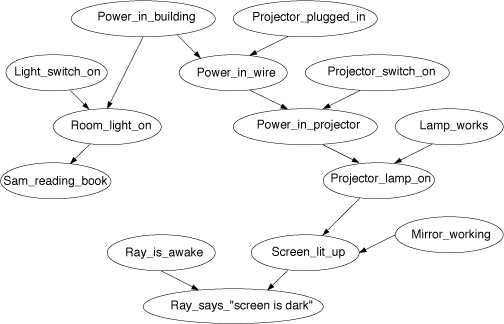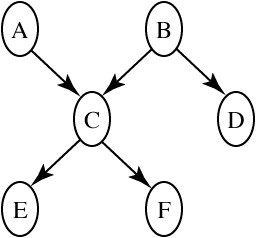6.8 Exercises

Exercise 6.1:
Using only the axioms of probability and the definition of conditional independence, prove Proposition 6.4.
Exercise 6.2:
Consider the belief network of Figure 6.21, which extends the electrical domain to include an overhead projector.Figure 6.21: Belief network for overhead projector

Answer the following questions about how knowledge of the values of some variables would affect the probability of another variable:

1. Can knowledge of the value of Projector_plugged_in affect your belief in the value of Sam_reading_book? Explain.
3. Can knowledge of Projector_plugged_in affect your belief in Sam_reading_book given that you have observed a value for Screen_lit_up? Explain.
4. Which variables could have their probabilities changed if just Lamp_works was observed?
5. Which variables could have their probabilities changed if just Power_in_projector was observed?
Exercise 6.3:
Represent the same scenario as in Exercise 5.8 using a belief network. Show the network structure and shade the observed nodes. Give all of the initial factors, making reasonable assumptions about the conditional probabilities (they should follow the story given in that exercise, but allow some noise).
Exercise 6.4:
Suppose we want to diagnose the errors school students make when adding multidigit binary numbers. Suppose we are only considering adding two two-digit numbers to form a three-digit number.

That is, the problem is of the form:

 A1 A0 + B1 B0 C2 C1 C0

,

where Ai, Bi, and Ci are all binary digits.

1. Suppose we want to model whether students know binary addition and whether they know how to carry. If they know how, they usually get the correct answer, but sometimes they make mistakes. If they don't know how to do the appropriate task, they simply guess.

What variables are necessary to model binary addition and the errors students could make? You must specify, in words, what each of the variables represents. Give a DAG that specifies the dependence of these variables.

2. What are reasonable conditional probabilities for this domain?
3. Implement this, perhaps by using the AISpace.org belief-network tool. Test your representation on a number of different cases.

You must give the graph, explain what each variable means, give the probability tables, and show how it works on a number of examples.

Exercise 6.5:
In this question, you will build a belief network representation of the Deep Space 1 (DS1) spacecraft considered in Exercise 5.10. Figure 5.14 depicts a part of the actual DS1 engine design.

Suppose the following scenario:

• Valves can be open or closed.
• A value can be ok, in which case the gas will flow if the valve is open and not if it is closed; broken, in which case gas never flows; stuck, in which case gas flows independently of whether the valve is open or closed; or leaking, in which case gas flowing into the valve leaks out instead of flowing through.
• There are three gas sensors that can detect gas leaking (but not which gas); the first gas sensor detects gas from the rightmost valves (v1...v4), the second gas sensor detects gas from the center valves (v5...v12), and the third gas sensor detects gas from the leftmost valves (v13...v16).
1. Build a belief-network representation of the domain. You only must consider the topmost valves (those that feed into engine e1). Make sure there are appropriate probabilities.
2. Test your model on some non-trivial examples.
Exercise 6.6:
Consider the following belief network:with Boolean variables (we write A=true as a and A=false as ¬a) and the following conditional probabilities:

 P(a) = 0.9 P(b) = 0.2 P(c|a,b) = 0.1 P(c|a,¬b) = 0.8 P(c|¬a,b) = 0.7 P(c|¬a,¬b) = 0.4
 P(d| b) = 0.1 P(d|¬b) = 0.8 P(e|c) = 0.7 P(e|¬c) = 0.2 P(f|c) = 0.2 P(f|¬c) = 0.9
1. Compute P(e) using VE. You should first prune irrelevant variables. Show the factors that are created for a given elimination ordering.
2. Suppose you want to compute P(e|¬f) using VE. How much of the previous computation can be reused? Show the factors that are different from those in part (a).
Exercise 6.7:
Explain how to extend VE to allow for more general observations and queries. In particular, answer the following:
1. How can the VE algorithm be extended to allow observations that are disjunctions of values for a variable (e.g., of the form X=a∨ X=b)?
2. How can the VE algorithm be extended to allow observations that are disjunctions of values for different variables (e.g., of the form X=a∨ Y=b)?
3. How can the VE algorithm be extended to allow for the marginal probability on a set of variables (e.g., asking for the marginal P(XY|e))?
Exercise 6.8:
In a nuclear research submarine, a sensor measures the temperature of the reactor core. An alarm is triggered (A=true) if the sensor reading is abnormally high (S=true), indicating an overheating of the core (C=true). The alarm and/or the sensor can be defective (S_ok=false, A_ok=false), which can cause them to malfunction. The alarm system can be modeled by the belief network of Figure 6.22.Figure 6.22: Belief network for a nuclear submarine

1. What are the initial factors for this network? For each factor, state what it represents and what variables it is a function of.
2. Show how VE can be used to compute the probability that the core is overheating, given that the alarm does not go off; that is, P(c |¬a).

For each variable eliminated, show which variable is eliminated, which factor(s) are removed, and which factor(s) are created, including what variables each factor is a function of. Explain how the answer can be derived from the final factor.

3. Suppose we add a second, identical sensor to the system and trigger the alarm when either of the sensors reads a high temperature. The two sensors break and fail independently. Give the corresponding extended belief network.
Exercise 6.9:
Let's continue Exercise 5.14.
1. Explain what knowledge (about physics and about students) a belief-network model requires.
2. What is the main advantage of using belief networks (over using abductive diagnosis or consistency-based diagnosis) in this domain?
3. What is the main advantage of using abductive diagnosis or consistency-based diagnosis compared to using belief networks in this domain?
Exercise 6.10:
In importance sampling, every non-observed variable is sampled; a full implementation of VE is not needed. Explain how to compute the probability of a sample given the evidence in this situation. [Hint: remember that it is possible to sample children as well as parents of observed variables.]
Exercise 6.11:
Consider the problem of filtering in HMMs.
1. Give a formula for the probability of some variable Xj given future and past observations. This should involve obtaining a factor from the previous state and a factor from the next state and combining them to determine the posterior probability of Xk. How can the factor needed by Xj-1 be computed without recomputing the message from Xj+1? [Hint: consider how VE, eliminating from the leftmost variable and eliminating from the rightmost variable, can be used to compute the posterior distribution for Xj.]
2. Suppose you have computed the probability distribution for each state S1, ..., Sk, and then you get an observation for time k+1. How can the posterior probability of each variable be updated in time linear in k? [Hint: you may need to store more than just the distribution over each Si.]
Exercise 6.12:
Consider the problem of generating a dynamic belief network given a particular discretization of time and given a representation in terms of transition time, and the state transition, as in Section 6.5.5. Suppose that there is an exponential distribution of how long each variable remains in a state and that the half-life of each variable value is specified. Give the dynamic belief network representation, assuming only a single transition in each time step.
Exercise 6.13:
Suppose you get a job where the boss is interested in localization of a robot that is carrying a camera around a factory. The boss has heard of variable elimination, rejection sampling, and particle filtering and wants to know which would be most suitable for this task. You must write a report for your boss (using proper English sentences), explaining which one of these technologies would be most suitable. For the two technologies that are not the most suitable, explain why you rejected them. For the one that is most suitable, explain what information is required by that technology to use it for localization:
1. VE (i.e., exact inference as used in HMMs),
2. rejection sampling, or
3. particle filtering.